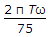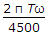# Mechanical Engineering - Strength of Materials

Exercise : Strength of Materials - Section 3
1.
The shear force and bending moment are zero at the free end of a cantilever beam, if it carries a
point load at the free end
point load at the middle of its length
uniformly distributed load over the whole length
none of the above
Explanation:
No answer description is available. Let's discuss.

2.
A rod is enclosed centrally in a tube and the assembly is tightened by rigid washers. If the assembly is subjected to a compressive load, then
rod is under compression
tube is under compression
both rod and tube are under compression
tube is under tension and rod is under compression
Explanation:
No answer description is available. Let's discuss.

3.
A bar of length L metres extends by l mm under a tensile force of P. The strain produced in the bar is
l/L
0.1 l/L
0.01 l/L
0.001 l/L
Explanation:
No answer description is available. Let's discuss.

4.
A shaft revolving at ω rad / s transmits torque (T) in N-m. The power developed is
T.ω watts
2 π Tω wattswattswatts